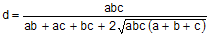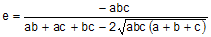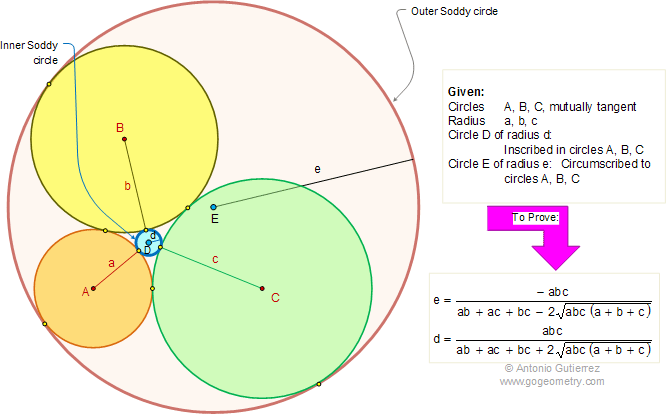Soddy Circles and Descartes Theorem, Three Tangent Circles. Inscribed and Circumscribed Circles, Radii. In the figure, circles A (radius a), B (radius b), and C (radius c) are mutually tangent. Circle D (radius d), called inner Soddy circle, is inscribed in circles A, B, and C. Circle E (radius e), called outer Soddy circle, is circumscribed to circles A, B, and C. Prove that (1), and (2). Post a comment.This formula is called the Descartes circle theorem since it was known to Rene Descartes (1596 - 1650). Frederic Soddy (1936) extended the result to tangent spheres. Typography Art in Motion using Mobile Apps, iPad. Click on the figure below. A video posted by Antonio Gutierrez (@gogeometry1) on Dec 30, 2016 at 2:54pm PST Recent Additions